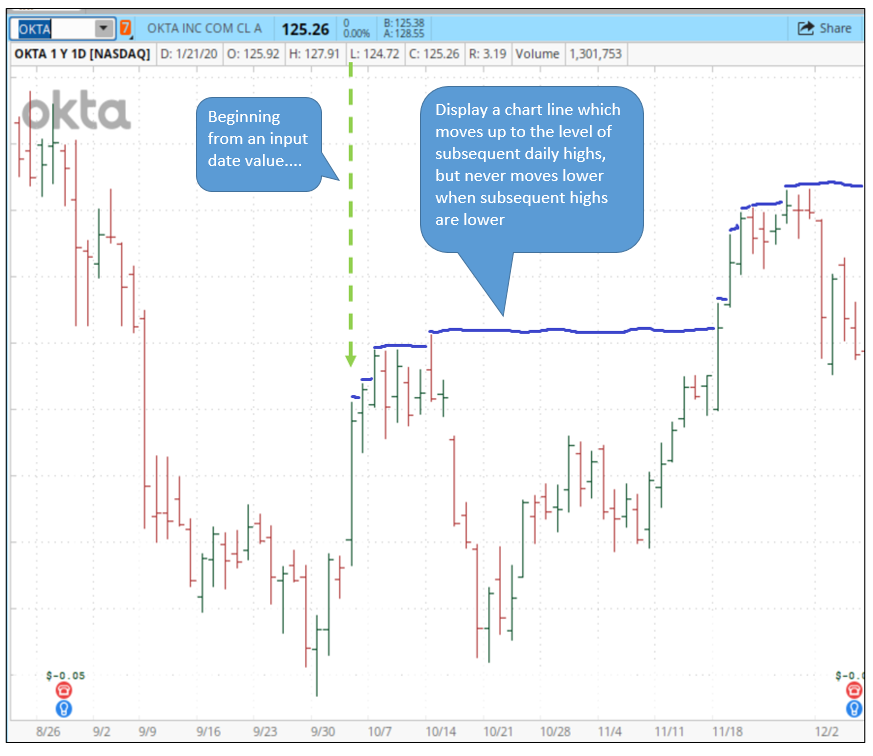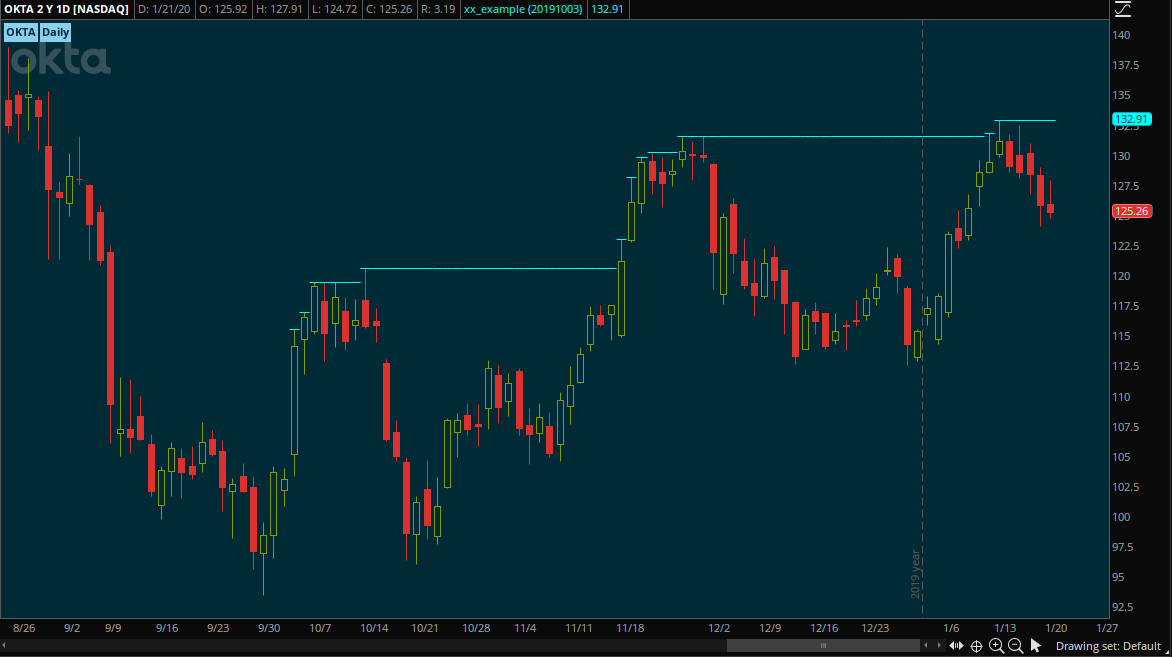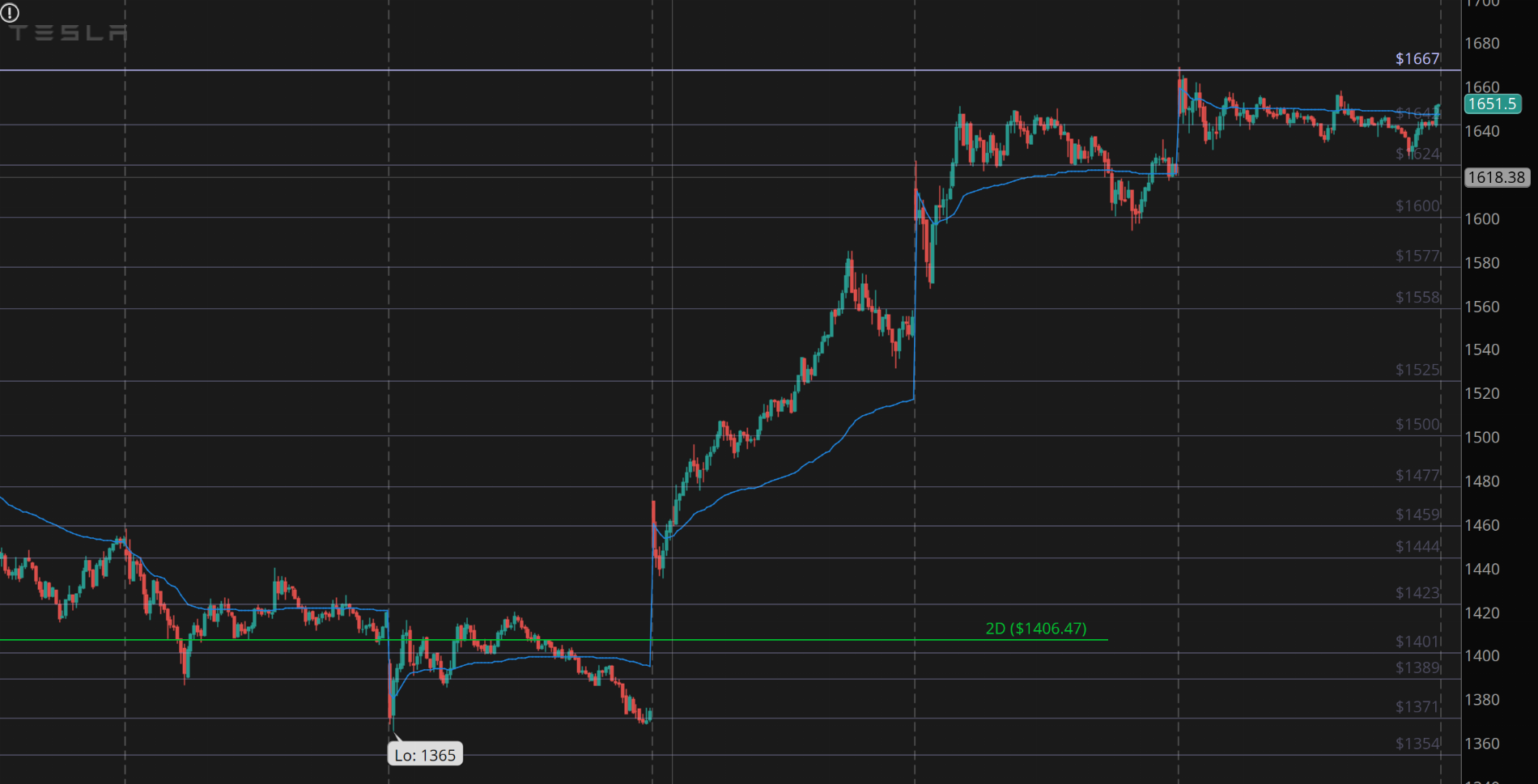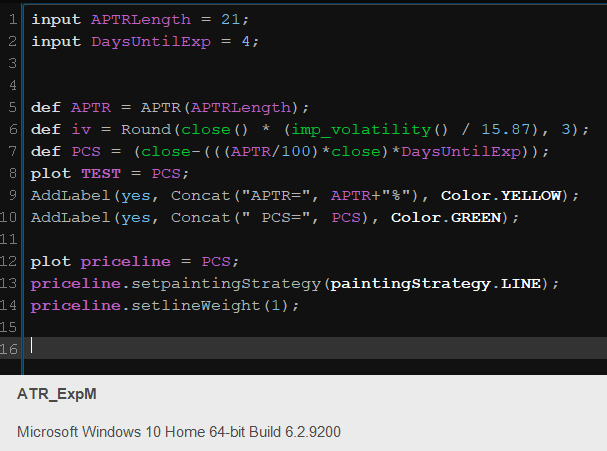# Horizontal Lines In ThinkOrSwim

#### Tom4235

##### New member
Hey, using thinkscript, how would you draw a horizontal line at high of day (HOD)? Just need the visual on my ThinkorSwim chart.

Update: more examples of how to plot horizontal lines are provided throughout this thread.

Here we go, horizontal line at HOD, use this on an intraday chart

Code:
``````plot highLine = highestAll(if isNaN(close[-1])
then high(period = "Day")
else Double.NaN);``````

Hi, this is a request for help with creating a study. I have simple scripting skills and I haven't been able to figure this out on my own. I would like to be able to plot a horizontal line on a chart from an inputted start date at that day's high. Then on subsequent days when the stock's high is higher, shift the horizontal line higher to that new high value. The line at the highs would move higher as the stock's prior high is exceeded, but the line would never move lower. Below is a link to a mock-up sketch on imgur.com that illustrates what I am trying to do. The way I want to use this is as a way to easily visualize how closely or not a stock is trending upward near its highs. Thanks very much.Ruby:
``````input StartDate = 20191003;
def hh = if GetYYYYMMDD() == StartDate then high else if high > hh then high else hh;
plot higherHigh = if GetYYYYMMDD() < StartDate then Double.NaN else hh;
higherHigh.SetPaintingStrategy(12);``````Last edited:
Pretty much the question folks. I have seen some codes from google for creating horizontal lines for the current price/position (in which they didnt work really) but i tried to mess with them to create this but been unsuccessful. Basically, if we have SPY on our chart, i want to see hortizontal lines at 307,307.5,308,308.5 that are close to the current price (50 instances?) for today, anyone have the script or interested in mocking something up?

Last edited:
Something like this would work

Code:
``````input level = 100;

plot a = level + 5;

plot b = level + 10;``````

•perdelta
plot my_line = 1; #change 1 to whatever static value or variable you want it to plot

how about the value of the first 1m candle each day opening? day.open types dont work

Code:
``````def mostRecentBar = !IsNaN(close) and IsNaN(close[-1]);
def mostRecentBarNumExtract = If mostRecentBar Then BarNumber() else Double.NaN;
def mostRecentBarNum = HighestAll(mostRecentBarNumExtract);
plot someStuff = If BarNumber() > (mostRecentBarNum - 5) Then high + 2 else Double.NaN;``````

EDIT: I see now this thread is 3+ months oldI would like to create multiple drawings of price line with price shown as the name, currently I am doing this manually and would like to know if there is a way to this via a script. Something like below for e.g TSLA

1] Input the list of price levels I would like to plot. (eg. 1600, 1650, 1700) I can edit the script itself and add these price levels.
2] Based on the number of prices I enter in the list it should iterate over the list and plot the price levels for all these price points.
3] It should also display the price name

Tthe price lines here were done manually, but looking for something similar which can be plotted all at once if given multiple levels.Code:
``````# Auto Horizontal Lines

input price1 = 130;
input price2 = 131;
input price3 = 132;

plot l1 = price1;
plot l2 = price2;
plot l3 = price3;``````

Adjust the inputs to the price levels you want to plot.

•perdelta and j05iah
Thanks Ben that helps . . . Just curious is there a way to define an array / list in thinkscript ? so that i can define an array with all the price levels and loop over it ?

Anyone have the code to add a "name" to the price level/line. Say I am plotting out 4-hour levels and want to identify it with a name to the right on the price line.

Last edited:
@Piper792 You can use the Price level drawing tool for that.

Yeah - I want to plot a series of levels and just input the price in the study. Of course with different prices. But exactly want to replicate price level

Code:
``````# 1hr UT
# 2hr UT
# 4hr UT
# Swing Pivot High

#Resistance
plot data1 = 1995.6; data1.SetDefaultColor(Color.Red);
plot data2 = 1995.6; data1.SetDefaultColor(Color.Red);
plot data3 = 1995.6; data1.SetDefaultColor(Color.Red);
plot data4 = 1995.6; data1.SetDefaultColor(Color.Red);

#Support
plot data5 = 1995.6; data1.SetDefaultColor(Color.Green);
plot data6 = 1995.6; data1.SetDefaultColor(Color.Green);
plot data7 = 1995.6; data1.SetDefaultColor(Color.Green);
plot data8 = 1995.6; data1.SetDefaultColor(Color.Green);``````

Im not the greatest at coding this stuff, but "PCS" in my code, i want a ongoing horizontal line that follows PCS. Kinda like a current close price line, but following my PCS price. Could someone please help me with this?First-time poster, long time lurker. I'm looking for a script to plot horizontal line at moving average crossover. I am NOT a coder by any means, I have attempted to modify/create a script to plot horizontal dashes or points at moving average crossovers. The original thinkorswim indicator plotted an up arrow at the crossover on the chart. I am wanting to have it display a horizontal dashed line or points instead. This is what I have tried but is not successful. I am sure it is not complete or I am missing something key. Any help would be much appreciated.

Code:
``````#
input price = close;
input length1 = 15;
input length2 = 30;
input averageType1 = AverageType.SIMPLE;
input averageType2 = AverageType.SIMPLE;
input crossingType = {default above, below};

def avg1 = MovingAverage(averageType1, price, length1);
def avg2 = MovingAverage(averageType2, price, length2);

plot signal = Crosses(avg1, avg2, crossingType == crossingType.above);

signal.DefineColor("Above", GetColor(6));
signal.DefineColor("Below", GetColor(7));
signal.AssignValueColor(if crossingType == crossingType.above then signal.Color("Above") else signal.Color("Below"));

signal.SetPaintingStrategy(if crossingType == crossingType.above
then PaintingStrategy.DASHES
else PaintingStrategy.DASHES);

signal.SetPaintingStrategy(PaintingStrategy.DASHES);
#-------------------------------------``````

Code:
``````#
input price = close;
input length1 = 15;
input length2 = 30;
input averageType1 = AverageType.SIMPLE;
input averageType2 = AverageType.SIMPLE;
input crossingType = {default above, below};

plot avg1 = MovingAverage(averageType1, price, length1);
plot avg2 = MovingAverage(averageType2, price, length2);
def sigtest;
switch(crossingType){
case above:
sigtest = avg1 crosses above avg2;
case below:
sigtest = avg1 crosses below avg2;
}

def sigline = if sigtest then (avg1+avg2)/2 else if sigtest and !sigtest then (avg1+avg2)/2 else sigline;
plot signal = sigline;
signal.DefineColor("Above", GetColor(6));
signal.DefineColor("Below", GetColor(7));
signal.AssignValueColor(if crossingType == crossingType.above then signal.Color("Above") else signal.Color("Below"));

signal.SetPaintingStrategy(PaintingStrategy.HORIZONTAL);

#-------------------------------------``````

Hello new friends!. This is a nice forum and am exited to be part of it. Kindly pardon me if I am posting in wrong place. But I will learn with your guidance. I am familiar with python and other languages now just facing some road blocks to learn thinkscript.

Can you please help me how I can make a think script which will plot price lines based on underlying symbol.

1. I want to draw price lines based on the stock.
2. The script should switch based on the stock that is in the display. I did below and it is some how not working.

Code:
``````AddLabel(yes, GetSymbol());

switch((GetSymbol()){

case "ZM" :
{ plot p_100 = 100;
plot P_207 = 207;
plot P_319 = 319;
plot P_429 = 429; }

case "AAPL":

{  Plot P_100 = 100;
Plot P_250 = 250;
Plot P_275 =275;  }
}``````

Last edited:

### Not the exact question you're looking for?

87k+ Posts
314 Online## The Market Trading Game Changer

Join 2,500+ subscribers inside the useThinkScript VIP Membership Club
• Exclusive indicators
• Proven strategies & setups
• Private Discord community
• Exclusive members-only content
• 1 full year of unlimited support

What is useThinkScript?

useThinkScript is the #1 community of stock market investors using indicators and other tools to power their trading strategies. Traders of all skill levels use our forums to learn about scripting and indicators, help each other, and discover new ways to gain an edge in the markets.

How do I get started?

We get it. Our forum can be intimidating, if not overwhelming. With thousands of topics, tens of thousands of posts, our community has created an incredibly deep knowledge base for stock traders. No one can ever exhaust every resource provided on our site.

If you are new, or just looking for guidance, here are some helpful links to get you started.

What are the benefits of VIP Membership?